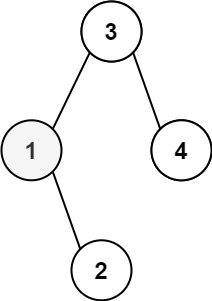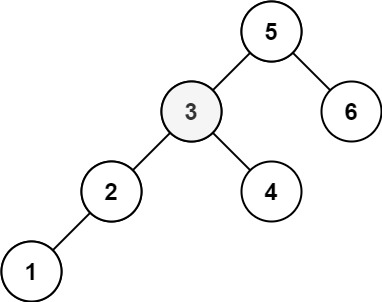# Kth Smallest Element in a BST

## 1: U-nderstand

Understand what the interviewer is asking for by using test cases and questions about the problem.

• Established a set (2-3) of test cases to verify their own solution later.
• Established a set (1-2) of edge cases to verify their solution handles complexities.
• Have fully understood the problem and have no clarifying questions.
• Have you verified any Time/Space Constraints for this problem?
• What is the type of tree we are working with?
• The input will be a binary search tree
• Can I assume the tree will be complete?
• No. A node may have less than 2 children
• Can I expect to receive an empty tree as input?
• No, the input tree can have 1-10000 nodes
• Will the kth item be within the bounds of n nodes?
• Yes, kth item is always inbound.
• What is the time and space constraints for this problem?
• `O(k)` time and `O(1)` space complexity without the recursive stack.
``````HAPPY CASE
Input: root = [3,1,4,null,2], k = 1
Output: 1````````````Input: root = [5,3,6,2,4,null,null,1], k = 3
Output: 3````````````EDGE CASE
Input: root = , k = 1
Output: 111``````

## 2: M-atch

Match what this problem looks like to known categories of problems, e.g. Linked List or Dynamic Programming, and strategies or patterns in those categories.

If you are dealing with Binary Trees some common techniques you can employ to help you solve the problem:

• Think about appropriate Tree Traversal: Pre-Order, In-Order, Post-Order, Level-Order
• Since we need to identify the kth item in sorted order. An in-order traversal will help us retrieve the nodes in sorted order and thereby give us the kth item.
• Store nodes within a HashMap to refer to later
• We don't need to access the nodes or their values after they have been processed, so this technique is not useful for this problem
• Using Binary Search to find an element
• We cannot use binary search for this problem because this does not help us identity the sorted order of the item in question.
• Applying a level-order traversal with a queue
• A level-order traversal is not helpful here. The level-order traversal does not help us with the sorted order.

## 3: P-lan

Plan the solution with appropriate visualizations and pseudocode.

General Idea: Recursive in-order traversal to help identify the kth node.

``````1) Create a helper function that processes in-order traversal while reducing k to help identify kth node.
a) Base Case: Check if the tree is empty or k is less than 0. If it is, return. We have completed search.
b) Call helper on left child
c) Reduce k and check if k is 0, if so set kth node and return
d) Call helper on right child
2) Create variable to hold kthNode
3) Call helper function on root
4) Return kthNode``````

⚠️ Common Mistakes

• Not recognizing that the input is a binary search tree
• Read the problem description carefully and clarify with your interviewer if you see this (or a different tree problem) in an interview
• Choosing the incorrect traversal type
• Try to walk through the problem by hand and see the order in which you are processing the nodes. This will clue you into the type of traversal necessary
• Suppose you chose pre-order traversal check - left - right. You will not be getting the smallest element first.
• Suppose you chose pre-order traversal left - right - check. You will not be getting the smallest element first.
• Not recognizing the need for a helper function
• To help reduce the number of parameters, remove the need for a return value, and retain memory.

## 4: I-mplement

Implement the code to solve the algorithm.

``````# Definition for a binary tree node.
# class TreeNode:
#     def __init__(self, val=0, left=None, right=None):
#         self.val = val
#         self.left = left
#         self.right = right
class Solution:
def kthSmallest(self, root: TreeNode, k: int) -> int:
# Create a helper function that processes in-order traversal while reducing k to help identify kth node
def helper(root: TreeNode):
nonlocal kthNode
nonlocal k

# Base Case: Check if the tree is empty or k is less than 0. If it is, return. We have completed search.
if not root or k < 0:
return

# Call helper on left child
helper(root.left)

# Reduce k and check if k is 0, if so set kth node and return
k -= 1
if k == 0:
kthNode = root.val
return

# Call helper on right child
helper(root.right)

# Create variable to hold kthNode
kthNode = 0

# Call helper function on root
helper(root)

# Return kthNode
return kthNode``````
``````class Solution {
// Create variable to hold kthNode
int ans = 0, i = 0;
public int kthSmallest(TreeNode root, int k) {
// Call helper function on root
inorder(root, k);
// Return kthNode
return ans;

}
// Create a helper function that processes in-order traversal while reducing k to help identify kth node
public void inorder(TreeNode root, int k) {
//  Base Case: Check if the tree is empty or k is less than 0. If it is, return. We have completed search.
if(root == null || k < 0) {
return;
}

// Call helper on left child
inorder(root.left, k);

// Reduce k and check if k is 0, if so set kth node and return
i++;
if(k==i){
ans = root.val;
return;
}

// Call helper on right child
inorder(root.right, k);
}
}``````

## 5: R-eview

Review the code by running specific example(s) and recording values (watchlist) of your code's variables along the way.

• Trace through your code with an input to check for the expected output
• Catch possible edge cases and off-by-one errors

## 6: E-valuate

Evaluate the performance of your algorithm and state any strong/weak or future potential work.

Assume `N` represents the number of nodes in binary search tree and `K` represents the kth node we want to find.

• Time Complexity: O(K)
• We only need visit each node along the path of the binary search tree until we reach the kth node.
• Space Complexity: O(1)
• O(1) when we do not account for recursive stack, but O(N) for space if we account for a recursion stack on a tree with a linked list type structure.